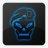# Plot daily pivots on RTH and/or ETH charts using only RTH Hours?

J

##### New member
Hello,

As the title implies, I am trying to plot daily pivots on both RTH and ETH charts, most notably on futures.

Unfortunately, I am having a very difficult time establishing the HLC for the RTH session. I seem to get the proper calculations with the code below, but only on a 10min chart. Anything else yields discrepancies.

I was hoping someone could help me streamline this. Any help or guidance is greatly appreciated.

And, to add just one bonus feature, would be the ability to have the user define the start and end time.

Thanks again

Code:
``````# Previous Intradays High, Low, Mean
# Mobius
# V01.12.2017 Desktop and Mobile
# Revised original code that uses SecondsFromTime() and SecondsTillTime(). Code now uses RegularTradingStart() and RegularTradingEnd() to bracket RTH. Works in Mobile Apps

def bar = barNumber();
def h = high;
def l = low;
def c = close;
def firstBar = if getTime() crosses above RegularTradingStart(GetYYYYMMDD()) and
!isNaN(close)
then bar
else double.nan;
def lastBar = if getTime() crosses above RegularTradingEnd(GetYYYYMMDD()) and
!isNaN(close)
then bar
else double.nan;
addVerticalLine(bar == HighestAll(firstBar), "first bar", color.cyan, curve.short_dash);
addVerticalLine(bar == HighestAll(lastBar), "last bar", color.cyan, curve.short_dash);
def bar_t1 = if !isNaN(firstBar)
then bar
else bar_t1;
def bar_t2 = if !isNaN(lastBar)
then bar
else bar_t2;
def prevFirstBar = if bar_t1 != bar_t1
then bar_t1
else prevFirstBar;
def prevLastBar = if bar_t2 != bar_t2
then bar_t2
else prevLastBar;
addVerticalLine(bar == HighestAll(prevFirstBar), "prev first bar", color.red, curve.short_dash);
addVerticalLine(bar == HighestAll(prevLastBar), "prev Last bar", color.red, curve.short_dash);
def hh = if bar == HighestAll(prevFirstBar)
then h
else if between(bar, highestAll(prevFirstBar), highestAll(LastBar)) and
h > hh
then h
else hh;
def hhBar = if h == hh and between(bar, highestAll(prevFirstBar), highestAll(LastBar))
then barNumber()
else double.nan;
def ll = if bar == HighestAll(prevFirstBar)
then l
else if between(bar, highestAll(prevFirstBar), highestAll(LastBar)) and
l < ll
then l
else ll;
def llBar = if l == ll and between(bar, highestAll(prevFirstBar), highestAll(LastBar))
then bar
else double.nan;

def LastC = if lastBar == bar then close else close;

DEF PrevDayHigh = if bar >= highestAll(hhBar)
then highestAll(if isNaN(c[-1])
then hh
else double.nan)
else double.nan;

DEF PrevDayLow = if bar >= HighestAll(llBar)
then highestAll(if isNaN(c[-1])
then ll
else double.nan)
else double.nan;

DEF PrevDayClose = if LastBar ==Barnumber()
then close
else double.nan;

def hl2bar = Floor((highestAll(hhbar) + highestAll(llBar)) / 2);
def hl2price = Round(((PrevDayHigh + PrevDayLow) / 2) / TickSize(), 0) * TickSize();

DEF PrevDayHL2 = if bar >= highestAll(HL2bar)
then highestAll(if !isNaN(c[-1])
then HL2price
else double.nan)
else double.nan;

# End Code Previous Days High, Low, Mean

# Start code forceIndex Pivot Point Calculations
def ppPrice = (PrevDayHigh + PrevDayLow +lastC) / 3;
def r1Price = ppPrice * 2 - PrevDayLow;
def r2Price = ppPrice + (PrevDayHigh - PrevDayLow);
def r3Price = ppPrice * 2 + (PrevDayHigh - 2 * PrevDayLow);
def r4Price = ppPrice * 3 + (PrevDayHigh - 3 * PrevDayLow);
def r5Price = ppPrice * 4 + (PrevDayHigh - 4 * PrevDayLow);
def s1Price = ppPrice * 2 - PrevDayHigh;

plot pp = if bar >= highestAll(bar)
then highestAll(if !isNaN(c[-1])
then ppPrice
else double.nan)
else double.nan;
plot R1 = if bar >= highestAll(HL2bar)
then highestAll(if !isNaN(c[-1])
then r1Price
else double.nan)
else double.nan;
plot R2 = if bar >= highestAll(HL2bar)
then highestAll(if !isNaN(c[-1])
then r2Price
else double.nan)
else double.nan;
plot R3 = if bar >= highestAll(HL2bar)
then highestAll(if !isNaN(c[-1])
then r3Price
else double.nan)
else double.nan;
plot R4 = if bar >= highestAll(HL2bar)
then highestAll(if !isNaN(c[-1])
then r4Price
else double.nan)
else double.nan;
plot R5 = if bar >= highestAll(HL2bar)
then highestAll(if !isNaN(c[-1])
then r5Price
else double.nan)
else double.nan;
plot S1 = if bar >= highestAll(HL2bar)
then highestAll(if !isNaN(c[-1])
then s1Price
else double.nan)
else double.nan;

#
# Formatting:
# Set color, line weight, style and hiding parameters
# for each pivot level
#
PP.SetDefaultColor(CreateColor(255, 102, 255));
PP.SetLineWeight(2);
PP.SetStyle(Curve.SHORT_DASH);
#
R1.SetDefaultColor(CreateColor(255, 102, 255));
R1.SetLineWeight(2);
R1.SetStyle(Curve.SHORT_DASH);
#
R2.SetDefaultColor(CreateColor(255, 102, 255));
R2.SetLineWeight(2);
R2.SetStyle(Curve.SHORT_DASH);
#
R3.SetDefaultColor(CreateColor(255, 102, 255));
R3.SetLineWeight(2);
R3.SetStyle(Curve.SHORT_DASH);
#
R4.SetDefaultColor(CreateColor(255, 102, 255));
R4.SetLineWeight(2);
R4.SetStyle(Curve.SHORT_DASH);
#
R5.SetDefaultColor(CreateColor(255, 102, 255));
R5.SetLineWeight(2);
R5.SetStyle(Curve.SHORT_DASH);
#
S1.SetDefaultColor(CreateColor(255, 102, 255));
S1.SetLineWeight(2);
S1.SetStyle(Curve.SHORT_DASH);``````

Last edited by a moderator:Plot a box for average daily price movement? Questions 3